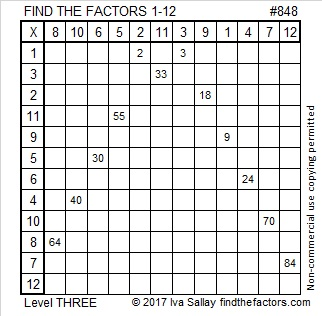# 848 and Level 3Print the puzzles or type the solution on this excel file: 12 factors 843-852

848 is a palindrome, and all but three of its factors are palindromes, too. (Single digit numbers are also palindromes.)

848 is the sum of two squares: 28² + 8² = 848

848 is the hypotenuse of a Pythagorean triple:

• 448-720-848, calculated from 2(28)(8), 28² – 8², 28² + 8²

844, 845, 846, 847, and 848 are the smallest five consecutive numbers whose square roots can be simplified.

• 848 is a composite number.
• Prime factorization: 848 = 2 × 2 × 2 × 2 × 53, which can be written 848 = 2⁴ × 53
• The exponents in the prime factorization are 4 and 1. Adding one to each and multiplying we get (4 + 1)(1 + 1) = 5 × 2 = 10. Therefore 848 has exactly 10 factors.
• Factors of 848: 1, 2, 4, 8, 16, 53, 106, 212, 424, 848
• Factor pairs: 848 = 1 × 848, 2 × 424, 4 × 212, 8 × 106, or 16 × 53
• Taking the factor pair with the largest square number factor, we get √848 = (√16)(√53) = 4√53 ≈ 29.1204396This site uses Akismet to reduce spam. Learn how your comment data is processed.